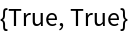Function Repository Resource:

# RuleReverse

Reverse a Rule or RuleDelayed expression

 ResourceFunction["RuleReverse"][rule] returns rule after swapping the left- and right-hand sides, while keeping undetermined variables on the left-hand side. ResourceFunction["RuleReverse"][rule,vars] forces vars as undetermined variables on the left-hand side of the output.

## Details

ResourceFunction["RuleReverse"] can be used on Rule and RuleDelayed expressions.

## Examples

### Basic Examples (2)

Reverse a Rule object:

 In:=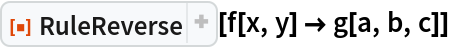Out=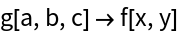Reverse a RuleDelayed object:

 In:=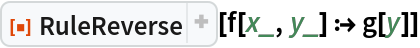Out=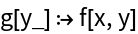### Properties and Relations (3)

Simple cases work like Reverse:

 In:=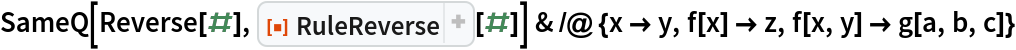Out=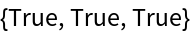With variable cases, blanks are forced to stay on the left-hand side:

 In:=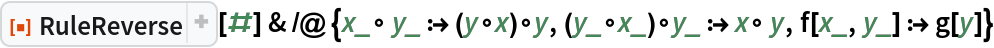Out=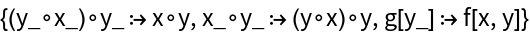RuleReverse preserves types when possible:

 In:=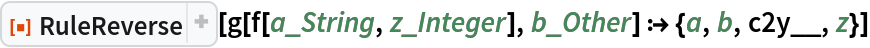Out=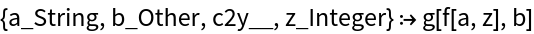### Possible Issues (2)

Applying RuleReverse twice does not act as the identity when variables are projected out:

 In:=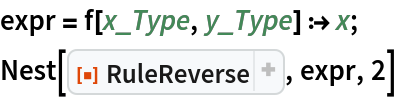Out=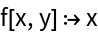In:=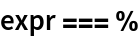Out=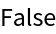This issue can be fixed by setting the extra vars argument:

 In:=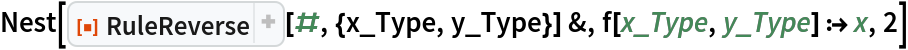Out=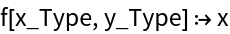In:=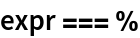Out=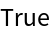If the right-hand side evaluates, repeated application might not act as the identity:

 In:=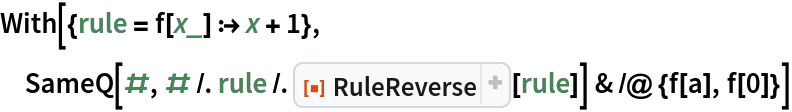Out=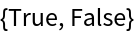This issue can be fixed by adding a Hold to the right-hand side:

 In:=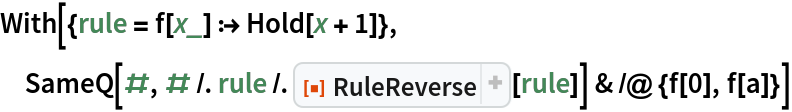Out=